# Precalculus : Find the Sum or Difference of Two Matrices

## Example Questions

1 3 Next →

### Example Question #21 : Find The Sum Or Difference Of Two Matrices

Let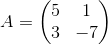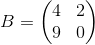Determine the sum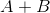.

Possible Answers: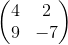DNE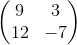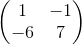Correct answer:Explanation:

Since the dimensions of the two matrices are equal the sum of the two matrices exists.

To find the sum, add each component entry from the first matrix to the same component entry of the second matrix.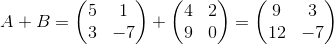### Example Question #22 : Find The Sum Or Difference Of Two Matrices

Let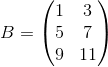Determine the sum.

Possible Answers: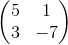DNE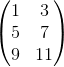Correct answer:

DNE

Explanation:

Because the dimensions of the two matrices are not equal

(A 2x2 matrix is not of the same dimension as a 3x2 matrix)

The sum does not exist.

### Example Question #21 : Find The Sum Or Difference Of Two Matrices

Simplify the following, reducing to one matrix: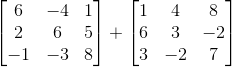Possible Answers: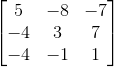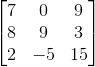Addition not possible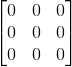Correct answer:Explanation:

To add matricies, first check to make sure they are the same size. If they are, then just add corresponding terms in the same spots in each matrix.

Since these are the same size we can add as follows.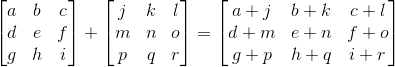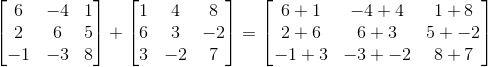### Example Question #24 : Find The Sum Or Difference Of Two Matrices

Find the sum if it exists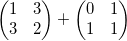Possible Answers: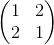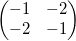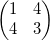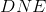Correct answer:Explanation:

Because the two matrices have the same dimension, the sum exists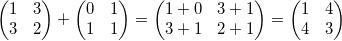### Example Question #25 : Find The Sum Or Difference Of Two Matrices

Sum the two matricies: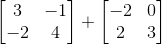Possible Answers: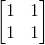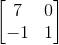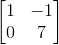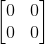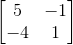Correct answer:Explanation:

To add matricies, simply add the corresponding terms. Thus,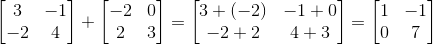### Example Question #26 : Find The Sum Or Difference Of Two Matrices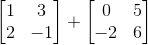Possible Answers: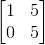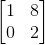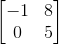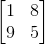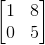Correct answer:Explanation:

To add two matrices, combine the corresponding numbers in the matrices: (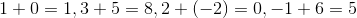). Then, put those answers in the corresponding spots in the answer matrix. Therefore, your answer is.

### Example Question #27 : Find The Sum Or Difference Of Two Matrices

Find the sum of the two matricesPossible Answers: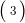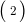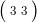Correct answer:Explanation:

Because the matrices do not have the same dimension, the sum does not exist.

1 3 Next →

### All Precalculus Resources